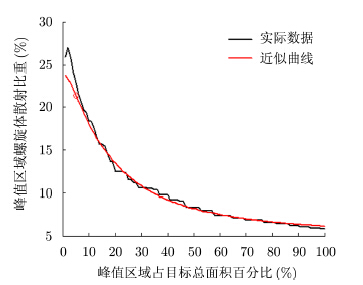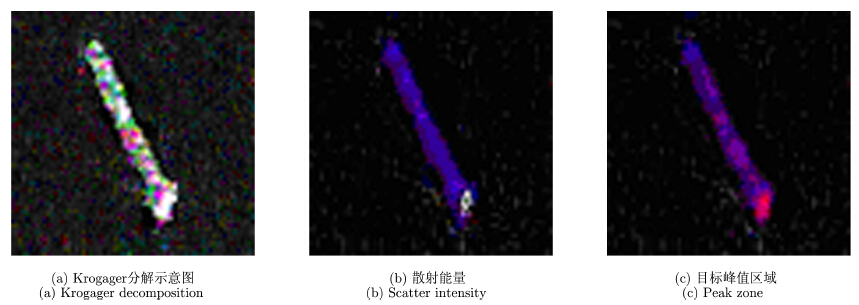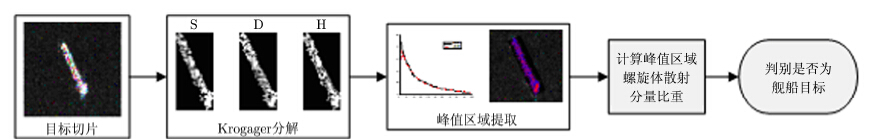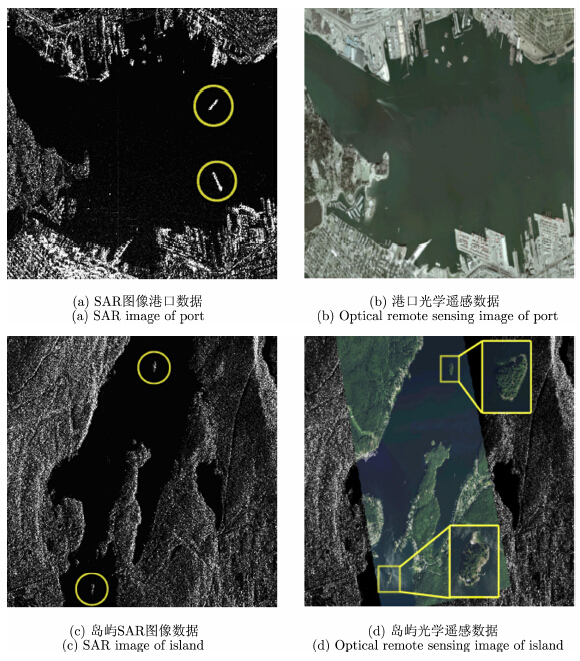﻿ 基于峰值区域的高分辨率极化<b>SAR</b>舰船目标特征分析与鉴别
«上一篇文章快速检索 高级检索

 雷达学报2015, Vol. 4Issue (3): 367-373  DOI: 10.12000/JR140930

### 引用本文 [复制中英文]

[复制中文]
Xu Cheng-bin, Zhou Wei, Cong Yu, et al.. Ship analysis and detection in high-resolution Pol-SAR imagery based on peak zone[J] Journal of Radars, 2015, 4(3): 367-373. DOI: 10.12000/JR14093.
[复制英文]

### 文章历史

Ship analysis and detection in high-resolution Pol-SAR imagery based on peak zone
Department of Electronic and Information Engineering, Naval Aeronautical and Astronautical University, Yantai 264001, China
Abstract: To deal with the problem of false alarm in the ship detection, a method base on proportion of spiral scattering in the peak zone is proposed. By comparing the proportion of spiral scattering in the peak zone, which is available from Krogager decomposition, the ships and interfering targets are identified and analyzed. The effectiveness of this method is justified with C-band full-polarization data from RADARSAT-2. The result show that this method can discriminate ships from interfering targets such as island, water-break, nautical platforms and bridges, thus reducing the false alarm rate of ship targets detection in SAR images.
Key words: Synthetic Aperture Radar (SAR)     Polarization decomposition     High-resolution     Ship detection
1 引言

2 Krogager极化相干分解

Krogager分解将对称的散射矩阵S分解为3个具有物理意义的相干分量之和，分别对应球散射、旋转角度为$\theta$的二面角散射和螺旋体散射：

 \begin{align} & {{S}_{(\mathrm{H,V})}}={{\text{e}}^{\text{j}\phi }}\left\{ {{\text{e}}^{\text{j}{{\phi }_{\mathrm{s}}}}}{{k}_{\text{S}}}{{S}_{\mathrm{sp}}}+{{k}_{\mathrm{D}}}{{S}_{\mathrm{di}(\theta )}}+{{k}_{\mathrm{H}}}{{S}_{\mathrm{he}(\theta )}} \right\} \\ & = {{\text{e}}^{\text{j}\phi }}\left\{ {{\text{e}}^{\text{j}{{\phi }_{\mathrm{s}}}}}{{k}_{\mathrm{S}}}\left[ \begin{matrix} 1 & 0 \\ 0 & 1 \\ \end{matrix} \right]+{{k}_{\mathrm{D}}}\left[ \begin{matrix} \cos 2\theta & \sin 2\theta \\ \sin 2\theta & -\cos 2\theta \\ \end{matrix} \right] \right. \\ & \left. \quad \ +{{k}_{\mathrm{H}}}{{\text{e}}^{\mp \text{j}2\theta }}\left[ \begin{matrix} 1 & \pm \text{j} \\ \pm \text{j} & -1 \\ \end{matrix} \right] \right\} \\ \end{align} (1)

 ${{S}_{n}}=k{{S}_{n-1}}={{k}^{n-1}}{{S}_{0}}$ (4)
 ${{P}_{n}}=t{{P}_{n-1}}={{t}^{n-1}}{{P}_{0}}$ (5)

 ${{P}_{0}}={\sum\limits_{i=1}^{m}{{{S}_{i}}{{P}_{i}}}}/{\sum\limits_{i=1}^{m}{{{S}_{i}}}}\;$ (9)图 4 最小误差近似变化曲线 Fig.4 Approximate variation curve with minimum error图 5 舰船目标峰值区域提取示意图 Fig.5 Extraction of ship target peak zone
4 基于峰值区域的舰船目标鉴别方法

SAR图像舰船检测中的干扰目标一般多为岛屿或者简单人造目标，岛屿目标强散射点多由于岛屿上的房屋、裸露的岩石等造成；简单人造目标强散射点主要归因于强烈的偶次散射机制。从目标整体上来分析，舰船目标与干扰目标都以平面散射为主，根据目标的实际结构各种散射机制所占比重会有所不同，但仍不能作为舰船目标与干扰目标的鉴别机理。例如表 1中所示数据为某岛屿目标与舰船目标之间Krogager分解后各散射分量比重。我们可以看到从整体散射机理来进行分析，舰船目标与某些干扰目标之间并没有明显差异，无法作为舰船目标与干扰目标的鉴别机理。表 1 目标Krogager分解各散射分量比重(%) Tab. 1 The proportion of each scattering with Krogager decomposition (%)

 ${{\rho }_{\mathsf{Q}}}={\sum\limits_{i=1}^{N}{{{k}_{\mathsf{Q}i}}}}/{\sum\limits_{i=1}^{N}{\left( {{k}_{\mathsf{S}i}}+{{k}_{\mathsf{D}i}}+{{k}_{\mathsf{H}i}} \right)}}\;\begin{matrix} , & \mathsf{Q}\in \left( \mathsf{S},\mathsf{D},\mathsf{H} \right) \\ \end{matrix}$ (10)图 6 舰船目标鉴别方法流程 Fig.6 The flow chart of ship target identification

5 实验与分析图 7 舰船目标和干扰目标示意图 Fig.7 The flow chart of ship targets and interfering targets表 2 RADARSAT-2数据中部分目标峰值区域螺旋体散射比重(%) Tab. 2 The proportion of target peak zone’s spiral scattering of parts of targets in RADARSAT-2 data (%)

6 结论

  邓云凯, 赵凤军, 王宇. 星载SAR 技术的发展趋势及应用浅析[J]. 雷达学报, 2012, 1(1): 1-10. Deng Yun-kai, Zhao Feng-jun, and Wang Yu. Brief analysis on the development and application of space borne SAR[J]. Journal of Radars, 2012, 1(1): 1-10.(1)  董江曼, 李应岐, 邓飚. SAR 图像舰船目标的特征识别[J]. 陕西师范大学学报(自然科学版), 2004, 32(S1): 203-205. Dong Jiang-man, Li Ying-qi, and Deng Biao. Ship targets recognition by ship's feature in SAR image[J]. Journal of Shaanxi Normal University (Natural Science Edition), 2004, 32(S1): 203-205.(1)  Kreithen D K, Halversen S D, and Owirka G J. Discriminating targets from clutter[J]. The Lincoln Laboratory Journal, 1993, 6(1): 25-52.(1)  张晰, 张杰, 纪永刚, 等. 基于结构特征的SAR船只类别识别能力分析[J]. 海洋学报, 2010, 32(1): 146-152. Zhang Xi, Zhang Jie, Ji Yong-gang, et al.. The capability analysis of ship classification by structure feature using SAR images[J]. Acta Oceanologica Sinica, 2010, 32(1): 146-152.(1)  Christoffersen T. Klassifisering av skip I SAR-bilder[R]. FFI/Notat-91/7046, Norwegian Defence Research Establishment, Kjeller, Norway, 1990.(1)  Osman H, Li P, Steven D B, et al.. Classification of ships in airborne SAR imagery using backprogation neural networks[C]. Proceedings of SPIE Symposium on Radar Processing, Technology, and Applications Ⅱ, San Diego, 1997, 3161: 126-136.(1)  陈琪, 陆军, 王娜, 等. 一种基于SAR图像鉴别的港口区域舰船目标新方法[J]. 宇航学报, 2011, 32(12): 2582-2588. Chen Qi, Lu Jun, Wang Na, et al.. An SAR images-based new method for ship discrimination in harbor region[J]. Journal of Astronautics, 2011, 32(12): 2582-2588.(1)  杨慧, 吴祥, 张建, 等. 基于HA-Wishar//αt分类的极化SAR图像船只检测[J]. 杭州电子科技大学学报, 2013, 33(6): 142-145. Yang Hui, Wu Xiang, Zhang Jian, et al.. Ship detection in polarimetric SAR imagery based on H/A/α-Wishart classification[J]. Journal of Hangzhou Dianzi University, 2013, 33(6): 142-145.(1)  肖顺平, 王雪松, 代大海, 等. 极化雷达成像处理及应用[M]. 北京: 科学出版社, 2013: 56-57. Xiao Shun-ping, Wang Xue-song, Dai Da-hai, et al.. Processing and Application of PolSAR Image[M]. Beijing: Science Press, 2013: 56-57.(1)  Krogager E and Czyz Z H. Properties of the sphere, deplane, helix decomposition[C]. Proceedings 3rd International Workshop on Radar Polarimetry (JIPR'95), Univ Nantes, France, 1995: 106-114.(1)  王超, 张红, 等. 全极化合成孔径雷达图像处理[M]. 北京: 科 学出版社, 2008: 75-76. Wang Chao, Zhang Hong, et al.. Image Processing of PolSAR Image[M]. Beijing: Science Press, 2008: 75-76.(1)  Lee Jong-sen and Pottier E. Polarimetric Radar Imaging: from Basics to Applications[M]. London, UK: Taylor and Francis Group, 2009: 154-155.(1)  高贵, 计科峰, 匡纲要, 等. 高分辨率SAR 图像目标峰值提取及峰值稳定性分析[J]. 电子与信息学报, 2005, 27(4): 561-565. Gao Gui, Ji Ke-feng, Kuang Gang-yao, et al.. Target peak extraction from high-resolution SAR image and peak's stability analysis[J]. Journal of Electronics & Information Technology, 2005, 27(4): 561-565.(1)  Xu Xiao-jian, Zhai Lai-juan, and Huang Ying. Subpixel processing for target scattering center extraction from SAR images[C]. International Conference on Signal Processing, Guilin, 2006: 2720-2723.(1)